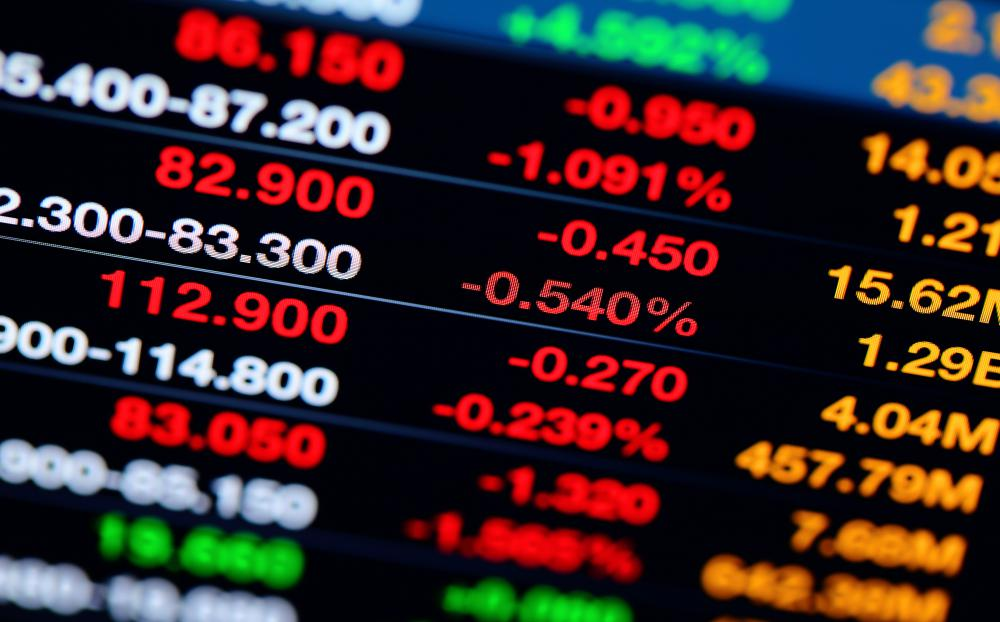# What is Stochastic Volatility?

A. Leverkuhn

A stochastic volatility model is a way to evaluate an investment in quantitative finance. The stochastic volatility model is used in looking at derivative securities, which are based on an original security or stock. Financial experts use stochastic volatility models to learn more about what is likely to happen with a derivative because of the properties of the security that it is based on.A stochastic volatility model is a way to evaluate an investment in quantitative finance.

In looking at how a derivate acts relative to the security that it is derived from, a stochastic volatility uses state variables. State variables are variables that identify changing attributes of a dynamic system, In thermodynamics, for example, state variables could include temperature and pressure. In finance, state variables could include things like industry volatility, market values, and event-driven speculative values, or other financial variables. The stochastic model is related to a “Black-Scholes” model where a specific formula is used in pricing European style options.The stochastic volatility model is used in looking at derivative securities, which are based on an original security or stock.

Stochastic models look at the way that volatility can change in a financial situation. One relevant trend that finance experts look at when using stochastic models for volatility is called a “volatility smile.” The volatility smile has to do with different states of derivatives, including in-the-money, at-the-money and out-of-the-money situations. All of these relate to the strike price of an option. More detailed information about the strike price, and when a derivative or option is in or out of the money, can be helpful to those who want to understand how stochastic volatility works. Essentially, the volatility smile shows that a security or derivative valuation can be different depending on the above condition of the strike price.

Several different types of stochastic volatility models are available to finance professionals, including the Heston model, the SABR (Stochastic Alpha, Beta, Rho) model, the GARCH (Generalized Autoregressive Conditional Heteroskedasticity) model, and the Chen model. When a user has selected the stochastic volatility model that suits their calculations the best, they will need to calibrate it against existing market data. The stochastic volatility will then provide a more accurate prediction for a derivative than if the calculation had just used a constant instead of running the volatility measure through this process.

There are many other terms that a student of finance needs to know in order to use stochastic processes for evaluating volatility. Skilled professionals understand the relation between each valuation method, and how to apply these methods to actual pricing models. Beginning with a solid grasp of derivatives and options, it’s easier for a student to become familiar with the basics of how these kinds of equations provide knowledge of a specific market situation.

## You might also Like